Next: CONSTRAINED FILTERS Up: Waveform applications of least Previous: DESIGN OF MULTICHANNEL FILTERS

# LEVINSON RECURSION

The Levinson recursion is a simplified method for solving normal equations. It may be shown to be equivalent to a recurrence relation in orthogonal polynomial theory. The simplification is Levinson's method is possible because the matrix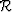has actually only N different elements when a general matrix could have N2 different elements.

Levinson developed his recursion with single time series in mind (the basic idea was presented in Section 3.3). It is very little extra trouble to do the recursion for multiple time series. Let us begin with the prediction-error normal equation. With multiple time series, unlike single time series, the prediction problem is changed if time is reversed. We may write both the forward and the backward prediction-error normal equations as one equation in the form of (36).

Since end effects play an important role, we will show how, when given the solution for 3-term filters,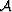and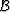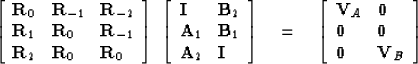(36)

to find the solution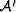and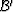four-term filters to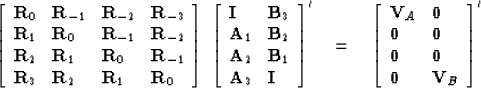(37)

by forming a linear combination ofand. This can be done by choosing constant matrices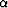and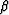in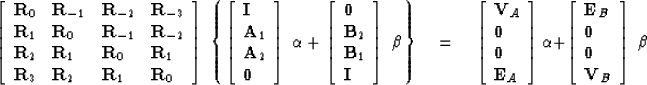(38)

Makeby choosingandso that the bottom element on the right-hand side of (38) vanishes. That is,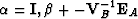.Makeby choosingandso that the top element on the right-hand side vanishes. That is,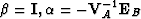.

Of course, one will want to solve more than just the prediction-error problem. We will also want to go from 3 x 3 to 4 x 4 in the solution of the filter problem with arbitrary right-hand side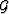.This is accomplished by choosing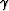in the following construction (39) so that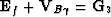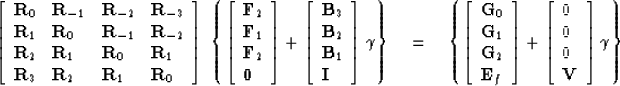(39)Next: CONSTRAINED FILTERS Up: Waveform applications of least Previous: DESIGN OF MULTICHANNEL FILTERS
Stanford Exploration Project
10/30/1997